Select Page

# Graph Coloring

Discrete Maths: Graph Coloring

Contents

Define various Graph Terminologies 1

Recall types of Graph 2

Recall Graph Operations 3

Recall Matrix representation of a Graph 4

Recall Isomorphic and Homeomorphic Graphs 7

Recall Connected Graph 9

Recall Eulerian and Hamiltonian Graph 10

Describe Planar Graph 12

Recall Kuratowski’s Graph 14

Describe Chromatic Number 15

Recall Chromatic Index 17

Describe Covering of a Graph 19

# Define various Graph Terminologies

Here are definitions for various graph terminologies:

1. Vertex/Node: A vertex, also known as a node, is a fundamental unit in a graph. It represents an entity or an object in the graph.
2. Edge: An edge is a connection or a link between two vertices in a graph. It represents a relationship or interaction between the entities represented by the vertices.
3. Directed Graph: Also known as a digraph, a directed graph is a graph in which the edges have a specific direction. In a directed graph, the edges have an arrow indicating the direction from one vertex to another.
4. Undirected Graph: An undirected graph is a graph in which the edges do not have any specific direction. The edges connect vertices without any indication of a one-way relationship.
5. Weighted Graph: A weighted graph is a graph in which each edge is assigned a weight or a value. The weight represents some meaningful quantity associated with the edge, such as distance, cost, or time.
6. Degree: The degree of a vertex in a graph is the number of edges incident to that vertex. In an undirected graph, the degree of a vertex is the number of edges connected to it. In a directed graph, the degree of a vertex is the sum of the in-degree and out-degree of that vertex.
7. Path: A path in a graph is a sequence of edges that connects a sequence of vertices. It represents a route or a series of steps taken to move from one vertex to another.
8. Cycle: A cycle in a graph is a path that starts and ends at the same vertex, without visiting any other vertex more than once. It represents a closed loop in the graph.
9. Connected Graph: A connected graph is a graph in which there is a path between every pair of vertices. In other words, there are no isolated vertices or disconnected components.
10. Complete Graph: A complete graph is a graph in which there is an edge between every pair of distinct vertices. In a complete graph with n vertices, there are n(n-1)/2 edges.
11. Bipartite Graph: A bipartite graph is a graph whose vertices can be divided into two disjoint sets such that there are no edges between vertices within the same set. In other words, the vertices can be partitioned into two independent sets.
12. Spanning Tree: A spanning tree of a graph is a subgraph that is a tree and connects all the vertices of the original graph. It contains all the vertices of the graph but only a subset of the edges.

These are some of the key terminologies used in graph theory to describe and analyze graphs.

# Recall types of Graph

Here are some types of graphs:

1. Undirected Graph: In an undirected graph, the edges do not have a specific direction. The relationship between vertices is symmetric, and the edges can be traversed in both directions.
2. Directed Graph (Digraph): In a directed graph, also known as a digraph, the edges have a specific direction. The relationship between vertices is asymmetric, and the edges represent one-way connections from one vertex to another.
3. Weighted Graph: In a weighted graph, each edge is assigned a weight or value. The weight represents a meaningful quantity associated with the edge, such as distance, cost, or time. Weighted graphs are used to model scenarios where edges have varying degrees of significance.
4. Complete Graph: A complete graph is a graph in which there is an edge between every pair of distinct vertices. In a complete graph with n vertices, there are n(n-1)/2 edges. Every vertex is directly connected to every other vertex in a complete graph.
5. Bipartite Graph: A bipartite graph is a graph whose vertices can be divided into two disjoint sets such that there are no edges between vertices within the same set. In other words, the vertices can be partitioned into two independent sets, and all edges connect vertices from one set to the other.
6. Connected Graph: A connected graph is a graph in which there is a path between every pair of vertices. There are no isolated vertices or disconnected components in a connected graph.
7. Disconnected Graph: A disconnected graph is a graph that is not connected. It consists of two or more disjoint components, where each component is a connected subgraph.
8. Tree: A tree is an undirected, connected, acyclic graph. It is a special type of graph where there is exactly one path between any two vertices. Trees have a hierarchical structure and do not contain any cycles or loops.
9. Directed Acyclic Graph (DAG): A directed acyclic graph, or DAG, is a directed graph that does not contain any directed cycles. In other words, it is a graph where there is no way to start at a vertex and follow a sequence of edges to return to the same vertex.
10. Planar Graph: A planar graph is a graph that can be drawn on a plane without any edges crossing each other. In other words, it is a graph that can be represented without any edge intersections.

These are some of the common types of graphs in graph theory. Each type has its own characteristics and applications in various fields of study.

# Recall Graph Operations

Here are some common operations that can be performed on graphs:

1. Add Vertex: This operation adds a new vertex to the graph. It involves creating a new node and connecting it to the existing vertices as desired.
2. Remove Vertex: This operation removes a vertex from the graph along with all its incident edges. It involves removing the node and updating the connections of the remaining vertices.
3. Add Edge: This operation adds a new edge between two existing vertices. It involves establishing a connection between the specified vertices.
4. Remove Edge: This operation removes an existing edge between two vertices. It involves disconnecting the specified vertices.
5. Degree of a Vertex: The degree of a vertex in a graph is the number of edges incident to that vertex. This operation calculates the degree of a given vertex.
6. Adjacency: The adjacency operation checks if there is an edge between two vertices. It determines whether two vertices are directly connected or not.
7. Breadth-First Search (BFS): BFS is a graph traversal algorithm that explores all the vertices of a graph in breadth-first order, starting from a specified vertex. It visits all the vertices at the same level before moving to the next level.
8. Depth-First Search (DFS): DFS is a graph traversal algorithm that explores all the vertices of a graph in depth-first order, starting from a specified vertex. It explores as far as possible along each branch before backtracking.
9. Shortest Path: This operation finds the shortest path between two vertices in a graph. It calculates the minimum number of edges or the minimum sum of weights required to reach from one vertex to another.
10. Minimum Spanning Tree (MST): MST is an operation that finds a tree that spans all the vertices of a graph while minimizing the total weight or cost. It is used to find the most efficient way to connect all the vertices in a graph.

These are some of the common operations performed on graphs. The choice of operation depends on the specific requirements and the type of graph being considered.

# Recall Matrix representation of a Graph

In a matrix representation of a graph, a two-dimensional matrix is used to represent the connections or edges between vertices. There are two commonly used matrix representations: the adjacency matrix and the incidence matrix.

In the adjacency matrix representation, a square matrix is used, where the rows and columns represent the vertices of the graph. The entry at position (i, j) in the matrix represents whether there is an edge between vertex i and vertex j. Typically, a value of 1 or a non-zero value indicates the presence of an edge, while a value of 0 or a zero value indicates the absence of an edge.

Example:

Consider a graph with four vertices labeled A, B, C, and D. The adjacency matrix representation of this graph would look like:

| A | B | C | D |

A | 0 | 1 | 1 | 0 |

B | 1 | 0 | 0 | 1 |

C | 1 | 0 | 0 | 1 |

D | 0 | 1 | 1 | 0 |

In this example, there is an edge between A and B, A and C, B and D, and C and D.

1. Incidence Matrix:

In the incidence matrix representation, a rectangular matrix is used, where the rows represent the vertices and the columns represent the edges of the graph. Each entry in the matrix indicates the relationship between a vertex and an edge. Typically, a value of 1 or a non-zero value indicates that the vertex is incident to the edge, while a value of 0 or a zero value indicates otherwise.

Example:

Consider a graph with four vertices labeled A, B, C, and D, and five edges labeled E1, E2, E3, E4, and E5. The incidence matrix representation of this graph would look like:

| E1 | E2 | E3 | E4 | E5 |

A | 1 | 0 | 1 | 1 | 0 |

B | 1 | 1 | 0 | 0 | 1 |

C | 0 | 1 | 1 | 0 | 1 |

D | 0 | 0 | 0 | 1 | 0 |

In this example, vertex A is incident to edges E1, E3, and E4, vertex B is incident to edges E1, E2, and E5, vertex C is incident to edges E2, E3, and E5, and vertex D is incident to edges E4.

Both adjacency matrix and incidence matrix representations have their advantages and are suitable for different scenarios, depending on the specific requirements of the graph algorithms or operations being performed.

3. Path Matrix:

The Path Matrix, also known as the Reachability Matrix, is a matrix representation of a graph that indicates whether there is a path between each pair of vertices in the graph. It is derived from the adjacency matrix by performing a series of matrix operations.

The Path Matrix is a square matrix where each entry at position (i, j) represents whether there is a path from vertex i to vertex j. Typically, a value of 1 or a non-zero value indicates the presence of a path, while a value of 0 or a zero value indicates the absence of a path.

To construct the Path Matrix, the matrix exponentiation operation is performed on the adjacency matrix. The exponentiation can be done using matrix multiplication or other efficient algorithms such as the Warshall’s algorithm.

Example:

Consider a graph with four vertices labeled A, B, C, and D. The adjacency matrix representation of this graph is:

| A | B | C | D |

A | 0 | 1 | 1 | 0 |

B | 0 | 0 | 0 | 1 |

C | 1 | 0 | 0 | 0 |

D | 0 | 0 | 1 | 0 |

To obtain the Path Matrix, we perform matrix exponentiation on the adjacency matrix. Let’s assume the exponentiation is done by matrix multiplication. After one iteration of matrix multiplication, we get the intermediate matrix:

| A | B | C | D |

A | 0 | 0 | 1 | 0 |

B | 0 | 0 | 0 | 1 |

C | 1 | 0 | 0 | 0 |

D | 0 | 0 | 1 | 0 |

After the second iteration of matrix multiplication, we obtain the final Path Matrix:

| A | B | C | D |

A | 1 | 0 | 1 | 1 |

B | 0 | 0 | 0 | 1 |

C | 1 | 0 | 1 | 1 |

D | 0 | 0 | 1 | 1 |

In this example, the Path Matrix indicates that there is a path from vertex A to vertex A, A to C, A to D, B to D, C to A, C to C, C to D, and D to C, and D to D. The absence of a path is represented by 0 in the matrix.

The Path Matrix is useful in graph algorithms and analysis, such as determining the reachability of vertices, finding transitive closure, and identifying connected components in a graph.

# Recall Isomorphic and Homeomorphic Graphs

Isomorphic Graphs:

Two graphs are said to be isomorphic if they have the same structure, meaning that there exists a one-to-one correspondence between their vertices such that the adjacency relationships are preserved. In other words, the nodes in one graph can be relabeled to match the nodes in the other graph while maintaining the same connectivity patterns.

Formally, two graphs G1 and G2 are isomorphic if there exists a bijective function f: V(G1) -> V(G2) such that for any two vertices u and v in G1, u and v are adjacent in G1 if and only if f(u) and f(v) are adjacent in G2.

Example:

Consider two graphs G1 and G2:

G1: A — B

| |

C — D

G2: X — Y

| |

Z — W

These two graphs are isomorphic because we can relabel the vertices in G1 to match the vertices in G2 as follows: A -> X, B -> Y, C -> Z, D -> W. This relabeling preserves the adjacency relationships, so the graphs are isomorphic.

Homeomorphic Graphs:

Two graphs are said to be homeomorphic if one can be obtained from the other by a series of vertex deletions, edge deletions, and edge contractions. In other words, homeomorphic graphs have the same topological structure, but the number of vertices and edges may differ.

Example:

Consider two graphs G1 and G2:

G1: A — B — C

| |

D — E

G2: X — Y — Z

These two graphs are homeomorphic because we can obtain G2 from G1 by deleting vertices D and E, and contracting the edge between B and C. This transformation preserves the topological structure of the graphs, so they are homeomorphic.

In summary, isomorphic graphs have the same structure, while homeomorphic graphs have the same topological structure but may differ in the number of vertices and edges.

# Recall Connected Graph

A connected graph is a graph in which there is a path between every pair of vertices. In other words, for any two vertices in a connected graph, there exists a sequence of edges that connects them.

Formally, a graph G is connected if, for every pair of vertices u and v in G, there exists a path from u to v.

A connected graph can be thought of as a single, unified component where every vertex is reachable from every other vertex. If a graph is not connected, it can be divided into multiple connected components.

Example:

Consider the following graph:

A — B

| |

C — D

This graph is connected because there is a path between every pair of vertices. For example, there is a path from A to B (A -> B), from B to C (B -> A -> C), from C to D (C -> A -> B -> D), and so on. Every vertex in the graph is reachable from every other vertex, so it is a connected graph.

# Recall Eulerian and Hamiltonian Graph

Eulerian Graph:

An Eulerian graph is a graph that contains an Eulerian cycle or an Eulerian path. An Eulerian cycle is a cycle in the graph that visits every edge exactly once and returns to the starting vertex, while an Eulerian path is a path that visits every edge exactly once but may or may not return to the starting vertex.

For a graph to be Eulerian, the following conditions must hold:

1. The graph must be connected.
2. Every vertex in the graph must have an even degree (even number of edges incident to it).

If a graph satisfies these conditions, it is said to be Eulerian, and it is possible to traverse all edges in the graph without lifting the pen (for an Eulerian cycle) or without lifting the pen until the last edge (for an Eulerian path).

Example:

Consider the following graph:

A — B

| / |

| / |

C — D

This graph is Eulerian because it satisfies the conditions for an Eulerian graph. It is connected, and every vertex (A, B, C, D) has an even degree (degree 2 for A and D, and degree 4 for B and C). Therefore, there exists an Eulerian cycle or an Eulerian path in this graph.

Hamiltonian Graph:

A Hamiltonian graph is a graph that contains a Hamiltonian cycle. A Hamiltonian cycle is a cycle in the graph that visits every vertex exactly once and returns to the starting vertex. In other words, it is a cycle that passes through all vertices of the graph.

Unlike an Eulerian cycle, a Hamiltonian cycle does not require visiting every edge exactly once, but rather every vertex exactly once.

Determining whether a graph is Hamiltonian is a challenging problem, and there is no simple criterion like the one for Eulerian graphs.

Example:

Consider the following graph:

A — B

| / |

| / |

C — D

This graph is not Hamiltonian because there is no cycle that visits every vertex exactly once. Although it is possible to traverse all vertices in a cycle (e.g., ABCDA), this is not a Hamiltonian cycle because it visits vertex C twice. Therefore, this graph is not Hamiltonian.

# Describe Planar Graph

A planar graph is a type of graph that can be embedded in a plane without any of its edges crossing each other. In other words, it can be drawn on a flat surface such as a piece of paper without any edge intersections.

Properties of Planar Graphs:

1. No Edge Crossings: In a planar graph, no two edges intersect each other. This property allows the graph to be drawn on a plane without any overlapping edges.
2. Faces: The plane in which a planar graph is embedded is divided into regions called faces. The outer face is the unbounded region, and the other faces are bounded by edges of the graph.
3. Euler’s Formula: For a connected planar graph with V vertices, E edges, and F faces, Euler’s formula states that V – E + F = 2. This formula relates the number of vertices, edges, and faces of a planar graph.
4. Planar Graph Subdivision: Any planar graph can be subdivided (split) into smaller planar graphs by adding extra vertices and edges.

Example:

Consider the following planar graph: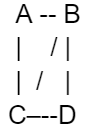This graph can be drawn on a plane without any edge crossings. It has four vertices (A, B, C, D), five edges (AB, AC, BC, BD, CD), and two faces (the interior of the graph and the exterior unbounded region). By applying Euler’s formula, we can verify that 4 – 5 + 2 = 1+2 = 3.

Note that not all graphs are planar. Non-planar graphs are those that cannot be drawn on a plane without edge crossings, such as a graph with edges that intersect each other.

Here are a few examples of planar graphs:

Tree: Any tree is a planar graph. A tree is a connected acyclic graph, and since it has no cycles, it can be drawn on a plane without any edge crossings.

Example: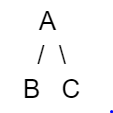Complete Graph: A complete graph, denoted as K_n, is a graph where every pair of distinct vertices is connected by an edge. For n ≤ 4, the complete graph is planar. However, for n ≥ 5, the complete graph becomes non-planar.

Example:

K4: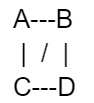Bipartite Graph: A bipartite graph is a graph whose vertex set can be partitioned into two disjoint sets such that every edge connects a vertex from one set to a vertex in the other set. Bipartite graphs are planar.

Example: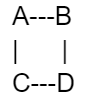Planar Subdivision of a Graph: Any graph that can be obtained from a planar graph by subdividing its edges is also planar.

Example: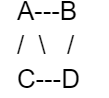Note that these are just a few examples of planar graphs. There are many more possible examples with varying numbers of vertices, edges, and complexities.

# Recall Kuratowski’s Graph

Kuratowski’s graph, also known as the Kuratowski subdivision or the Kuratowski subgraph, is a special type of graph used in graph theory to define planarity. It is named after the Polish mathematician Kazimierz Kuratowski.

Kuratowski’s graph is a non-planar graph that serves as a counterexample to the planarity of a graph. It is often denoted as K5 (the complete graph on five vertices) or K3,3 (a bipartite graph with three vertices on each side).

Kuratowski’s graph consists of either five vertices and 10 edges (K5) or six vertices and nine edges (K3,3). It cannot be embedded in a plane without edge crossings, which violates the definition of planar graphs.

The graph K5: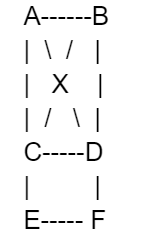The graph K3,3: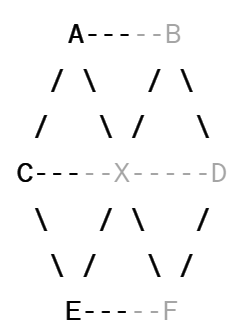Kuratowski’s graph plays a significant role in the theory of planar graphs. According to Kuratowski’s theorem, a graph is non-planar if and only if it contains a subgraph that is a subdivision of K5 or K3,3. This theorem provides a criterion to determine whether a graph is planar or not. If a graph does not contain Kuratowski’s graph as a subgraph, it is guaranteed to be planar.

# Describe Chromatic Number

The chromatic number of a graph is the minimum number of colors needed to color the vertices of the graph such that no adjacent vertices have the same color. It is denoted by χ(G), where G is the graph.

In other words, the chromatic number represents the minimum number of distinct colors required to color each vertex of the graph in such a way that no two adjacent vertices have the same color.

Key points about the chromatic number:

1. The chromatic number is always a positive integer.
2. It is a measure of the “colorability” of a graph.
3. The chromatic number is a property of the graph and can vary from one graph to another.
4. It is influenced by the structure and connectivity of the graph.
5. The chromatic number is always greater than or equal to 1.
6. The chromatic number of a graph can be determined using various algorithms and heuristics.

The concept of the chromatic number is important in various areas, including graph theory, computer science, and scheduling problems.

Determining the chromatic number of a graph is often a challenging task and can have practical implications in real-world applications, such as scheduling tasks with resource constraints or assigning frequencies to wireless communication channels.

For example, consider a graph with four vertices arranged in a cycle: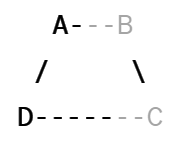In this case, the chromatic number of the graph is 2. We can color vertex A with color 1 and vertices B, C, and D with color 2. This coloring satisfies the condition that no two adjacent vertices have the same color. Therefore, χ(G) = 2 for this graph.

Here are a few more examples:

1. Complete Graph: A complete graph with n vertices, denoted as Kn, has all possible edges between its vertices. The chromatic number of a complete graph is always equal to the number of vertices, χ(Kn) = n. For example, the chromatic number of K3 (a triangle) is 3, and the chromatic number of K4 (a complete graph with 4 vertices) is 4.
2. Bipartite Graph: A bipartite graph is a graph whose vertices can be divided into two disjoint sets such that no two vertices within the same set are adjacent. Bipartite graphs have a chromatic number of 2, χ(G) = 2. For example, consider a bipartite graph with two sets of vertices A = {A1, A2} and B = {B1, B2}. The edges only exist between vertices of different sets (A and B). In this case, the chromatic number is 2.
3. Cycle Graph: A cycle graph, denoted as Cn, is a graph with n vertices arranged in a cycle. The chromatic number of a cycle graph is 2 for even values of n and 3 for odd values of n. For example, the chromatic number of C4 (a square) is 2, and the chromatic number of C5 (a pentagon) is 3.
4. Tree Graph: A tree is an acyclic connected graph. Every tree graph is a bipartite graph, so its chromatic number is 2, χ(G) = 2. For example, consider a tree graph with 5 vertices arranged in a hierarchical structure. The chromatic number of this tree graph is 2.
5. Grid Graph: A grid graph is formed by arranging vertices in a regular grid pattern, like a chessboard. The chromatic number of a grid graph depends on the size of the grid. For an m × n grid graph, the chromatic number is 2 if either m or n is even, and 3 if both m and n are odd. For example, a 3 × 3 grid graph has a chromatic number of 3.

These are just a few examples, and the chromatic number can vary for different types of graphs. The determination of the chromatic number for a given graph can involve various algorithms and techniques.

# Recall Chromatic Index

The chromatic index of a graph is the minimum number of colors needed to properly color the edges of the graph such that no two adjacent edges have the same color. It is denoted as χ'(G). The concept of the chromatic index is related to edge coloring in graphs.

Some examples of the chromatic index of certain graphs are:

1. Complete Graph: The chromatic index of a complete graph with n vertices, denoted as Kn, is n-1. This means that for a complete graph, every edge can be assigned a unique color.
2. Cycle Graph: The chromatic index of a cycle graph, denoted as Cn, is 2 for n ≥ 3. This means that for a cycle graph, only two colors are needed to properly color the edges, and no two adjacent edges have the same color.
3. Bipartite Graph: The chromatic index of a bipartite graph is equal to its maximum degree. A bipartite graph is a graph whose vertices can be divided into two disjoint sets such that no two vertices within the same set are adjacent.
4. Complete Bipartite Graph: The chromatic index of a complete bipartite graph, denoted as Kp,q, where p and q are the number of vertices in the two sets, is equal to the maximum degree of the graph.
5. Grid Graph: The chromatic index of a grid graph, formed by arranging vertices in a regular grid pattern, depends on the size of the grid. For an m × n grid graph, the chromatic index is equal to the maximum degree of the graph.

The determination of the chromatic index for a given graph can involve various algorithms and techniques, similar to determining the chromatic number of a graph.

Difference between chromatic number and Chromatic Index

Here’s a tabular comparison between the chromatic number and chromatic index:

 Chromatic Number Chromatic Index Definition Minimum number of colors to color vertices Minimum number of colors to color edges Denoted as χ(G) or χv(G) χ'(G) Focus Vertices Edges Purpose Ensure no adjacent vertices have the same color Ensure no adjacent edges have the same color Examples In a complete graph (Kn), χ(G) = n In a complete graph (Kn), χ'(G) = n-1 In a cycle graph (Cn) with n ≥ 3, χ(G) = 2 In a cycle graph (Cn) with n ≥ 3, χ'(G) = 2

This table summarizes the key differences between the chromatic number and chromatic index, including their definitions, notation, focus, purpose, and examples.

# Describe Covering of a Graph

In graph theory, the covering of a graph refers to the process of selecting a subset of vertices or edges in such a way that every vertex or edge in the graph is incident to at least one selected vertex or edge. The goal is to cover the entire graph with the minimum number of selected elements.

There are two main types of coverings in graph theory:

1. Vertex Cover: A vertex cover of a graph is a subset of vertices such that every edge in the graph is incident to at least one selected vertex. In other words, for every edge (u, v), either vertex u or vertex v (or both) must be in the vertex cover. The minimum size of a vertex cover is known as the vertex cover number.
2. Edge Cover: An edge cover of a graph is a subset of edges such that every vertex in the graph is incident to at least one selected edge. In other words, for every vertex u, there must be at least one edge incident to u in the edge cover. The minimum size of an edge cover is known as the edge cover number.

The concept of covering in graph theory is useful in various applications, such as network design, resource allocation, and optimization problems. Finding the minimum vertex cover or edge cover in a graph is a well-studied problem and has practical implications in various real-world scenarios.

Example 1: Vertex Cover

Consider the following graph: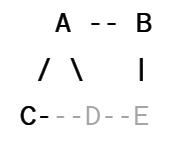A vertex cover of this graph can be {A, D}, where vertices A and D are selected. With this vertex cover, every edge in the graph is incident to at least one selected vertex. Other valid vertex covers for this graph could be {B, C} or {B, D, E}, among others.

Example 2: Edge Cover

Consider the following graph:An edge cover of this graph can be {AB, AC, BC}, where edges AB, AC, and BC are selected. With this edge cover, every vertex in the graph is incident to at least one selected edge. Other valid edge covers for this graph could be {AB, CD} or {BC, DE}, among others.

In both examples, the goal is to select the minimum number of vertices or edges to cover the entire graph. The specific vertex or edge selection may vary, but the objective is to ensure that every vertex or edge in the graph is incident to at least one selected element.